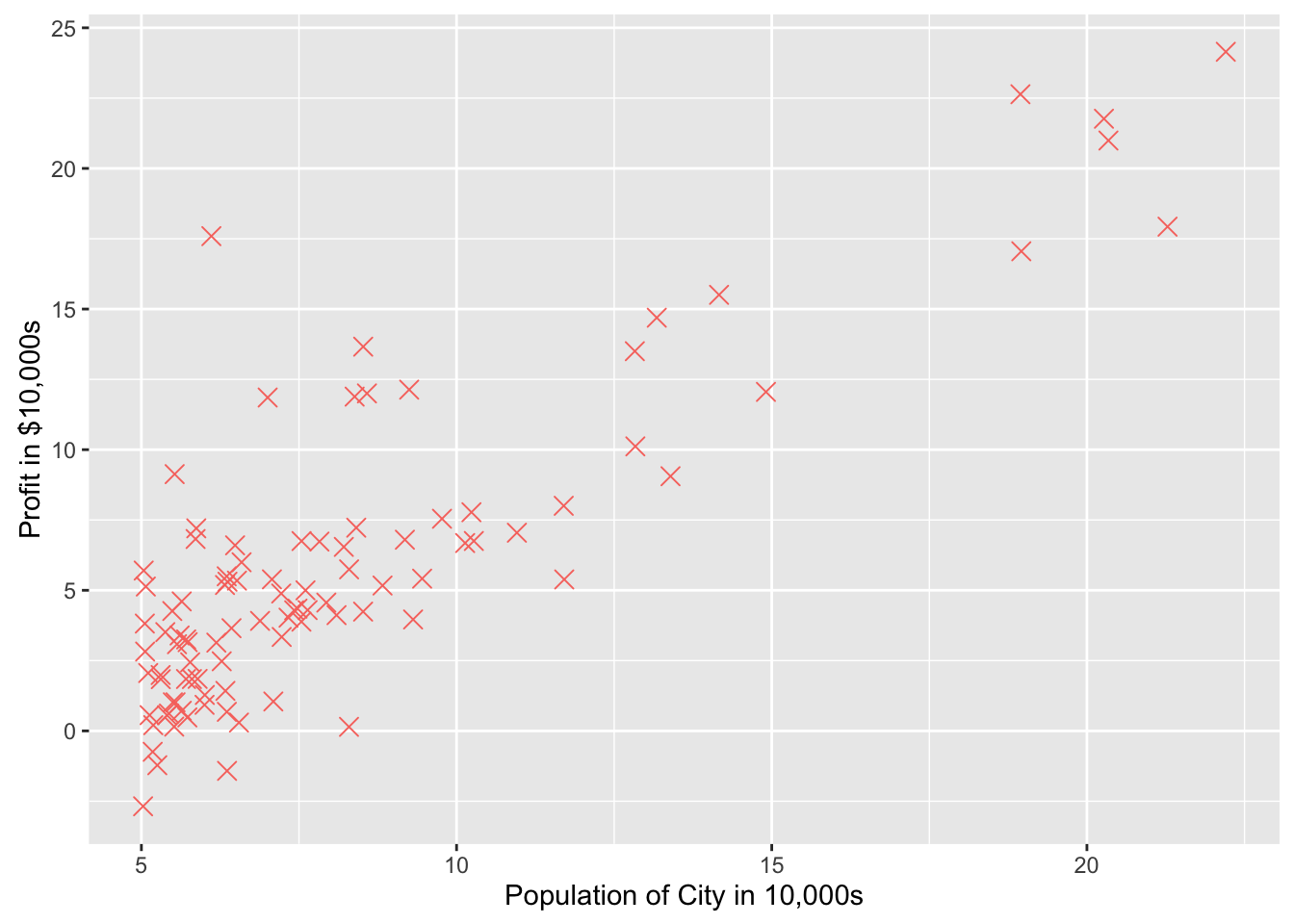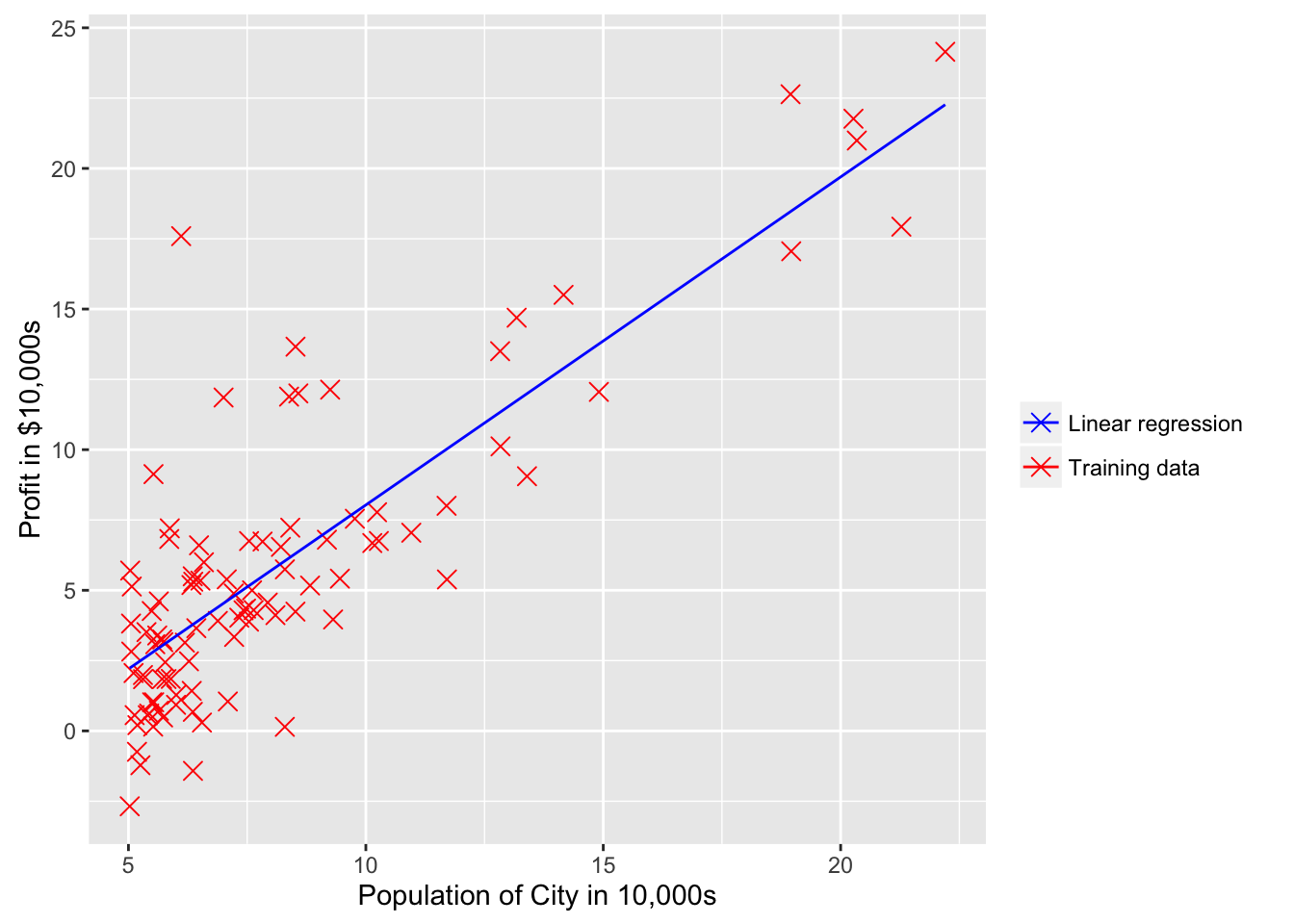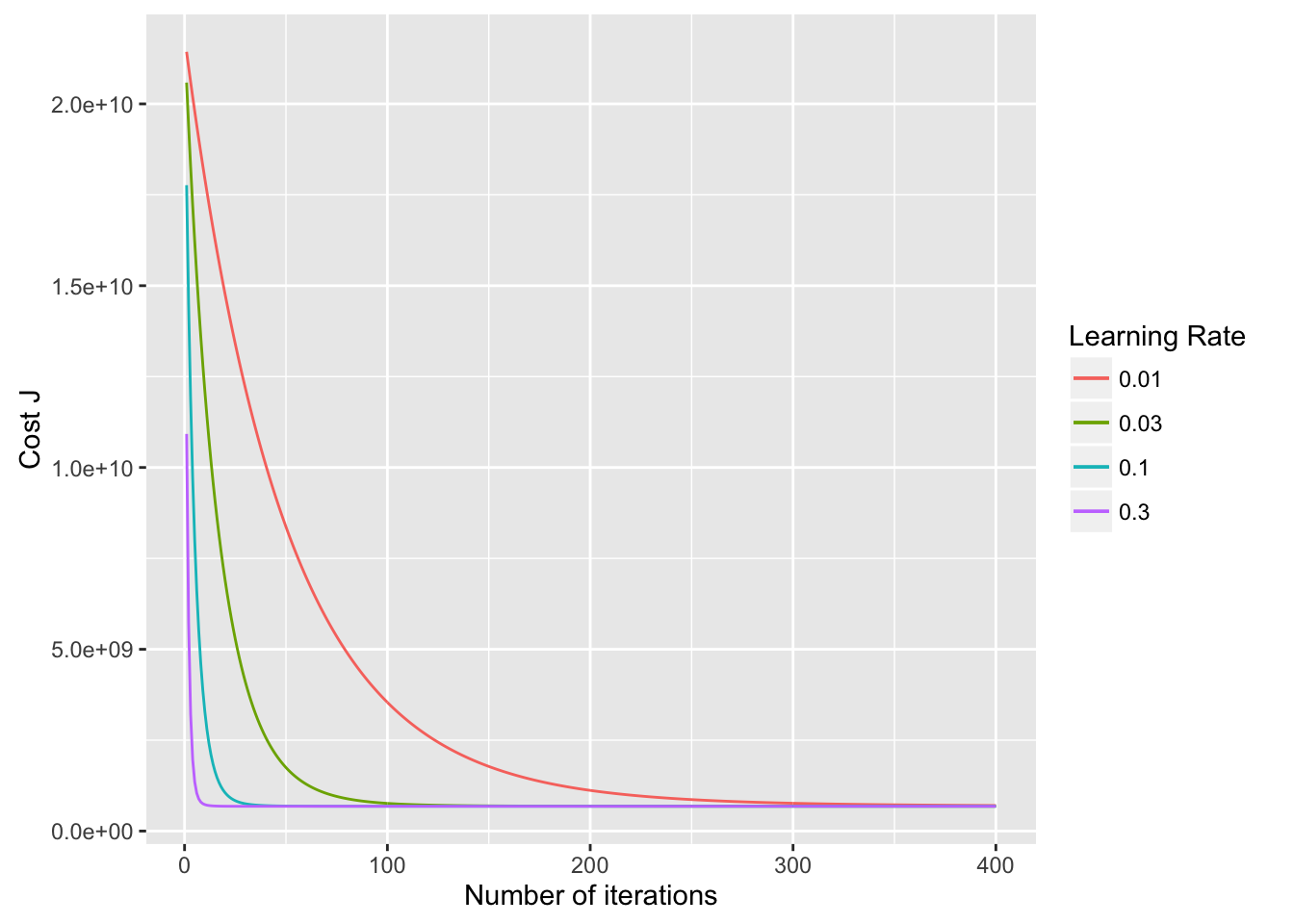# ML With R (1): Linear Regression

Code in R for Coursera-ML and CMPUT466/551 in University of Alberta

This post refers to programming exercise 1 in Coursera-ML. All the code can be found in this repo.

setwd("~/Coding/R/CMPUT466/mlclass-ex1")
library(ggplot2)
library(MASS)

### Linear regression with one variable

#### Plotting the Data

Use a scatter plot to visualize the data.

data <- read.csv("ex1data1.txt", header=FALSE)
ggplot(data, aes(V1, V2, col="Training data")) +
geom_point(shape=I(4), size=I(3)) +
labs(x="Population of City in 10,000s", y="Profit in $10,000s") + scale_color_discrete(guide=FALSE)#### Gradient Descent Compute the cost function: $J(\theta)=\frac1{2m}\sum_{i=1}^m(h_\theta(x^{(i)})-y^{(i)})^2$ where the hypothesis $$h_\theta(x)$$ is given by the linear model $h_{\theta)}(x)=\theta^T x$ computeCost = function(X, y, theta) { m = length(X) return(sum(((X %*% theta) - y)^2)/(2*m)) } X <- as.matrix(data$V1)
X <- cbind(1, X)
y <- as.matrix(data$V2) theta <- matrix(0, 2, 1) computeCost(X, y, theta) ##  16.03637 Use batch gradient descent algorithm to minimize cost function. In each iteration: $\theta_j = \theta_j - \alpha\frac1m\sum_{i=1}^m(h_\theta(x^{(i)}) - y^{(i)})x_j^{(i)}$ iterations <- 1500 alpha <- 0.01 m <- length(y) J_history <- matrix(0, iterations, 1) for(i in 1:iterations) { theta <- theta - alpha/m*t(X) %*% (X %*% theta - y) J_history[i] <- computeCost(X, y, theta) } ggplot(data, aes(V1, V2, col="Training data")) + geom_point(shape=I(4), size=I(3)) + labs(x="Population of City in 10,000s", y="Profit in$10,000s") +
geom_line(aes(X[,2], X %*% theta, col="Linear regression")) +
scale_color_manual(name="", values=c("Blue", "Red"))### Linear regression with multiple variables

#### Feature Normalization

When features differ by orders of magnitude, performing feature scaling can make gradient descent converge much more quickly. The scale function in R can be useful in this task.

data <- read.csv("ex1data2.txt", header=FALSE)
X <- as.matrix(data[,c("V1", "V2")])
y <- as.matrix(data$V3) m <- length(y) X <- scale(X) mu <- attr(X, "scaled:center") sigma <- attr(X, "scaled:scale") X <- cbind(1, X) #### Gradient Descent Computing the cost function and the gradient descent algorithm are similar to the univariate case. Four different learning rates are selected to see the rate of convergence. alpha = c(0.01, 0.03, 0.1, 0.3) num_iters = 400 theta = matrix(0, 3, 4) J_history <- matrix(0, num_iters, 4) for(i in 1:num_iters) { for(j in 1:4) { theta[,j] <- theta[,j] - alpha[j]/m*t(X) %*% (X %*% theta[,j] - y) J_history[i, j] <- computeCost(X, y, theta[,j]) } } df <- data.frame(x=1:num_iters, y=J_history) ggplot(df, aes(x)) + labs(x="Number of iterations", y="Cost J") + geom_line(aes(y=y.1, col="0.01")) + geom_line(aes(y=y.2, col="0.03")) + geom_line(aes(y=y.3, col="0.1")) + geom_line(aes(y=y.4, col="0.3")) + scale_color_discrete(name="Learning Rate")Use $$\theta$$ that we’ve found to predict the price of a house with $$1650$$ square feet and 3 bedrooms. Remember to do the feature normalization first! X_predict <- (c(1650., 3.) - mu)/sigma price <- c(1, X_predict) %*% theta price ## [,1] [,2] [,3] [,4] ## [1,] 289314.6 293150 293081.5 293081.5 #### Normal Equations The closed-form solution to linear regression is: $\theta=(X^T X)^{-1}X^T y$ data <- read.csv('ex1data2.txt', header=FALSE) X <- as.matrix(data[, c("V1", "V2")]) y <- as.matrix(data$V3)
m <- length(y)

X <- cbind(1, X)

theta = ginv(t(X) %*% X, tol=0) %*% t(X) %*% y

price <- c(1, 1650, 3) %*% theta
price
##          [,1]
## [1,] 293081.5

The predicted price is almost the same as the price obtained using gradient descent.